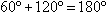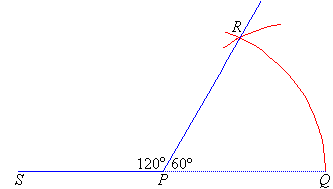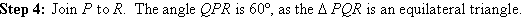# How to construct 120 degree and 60 degree angle?

• 1
steps for construction of 60 degree and 120 degree angles:-
• draw a line RQ.
• mark a point say P.
• with P as the centre make an arc with compass(rounder).
• mark the end points of the arc as A and B.
• with as the centre with same radius make a cut say S on the arc.
• make a straight line passing through P and S .
• thus 60 degree angle is formed.
• now with the same radius make a cut from point S to point V.
• make a straight line passing through P and V.
• thus 120 degree angle is formed.
• -3
Constructing a 120º Angle

We know that:This means that 120º is the supplement of 60º.  Therefore, to construct a 120º angle, construct a 60º angle and then extend one of its arms as shown below.Constructing a 60º Angle

We know that the angles in an equilateral triangle are all 60º in size.  This suggests that to construct a 60º angle we need to construct an equilateral triangle as described below.

Step 1:  Draw the arm PQ.
Step 2:  Place the point of the compass at P and draw an arc that passes through Q.
Step 3:  Place the point of the compass at Q and draw an arc that passes through P.  Let this arc cut the arc drawn in Step 2 at R.• -2
Hi! Lohitaksh,
​Here Are The Steps Of Construction:
1. Draw a line segment and mark a point B on it.
2. Set the compass to a specific radius and with B as centre, draw a semi-circular arc and label the arc as XY.
3. With Y as centre, having the compass set into the same radius as in step 2, draw an arc cutting/intersecting arc XY at C.
4. Join C with B.
5. 60o Angle will be constructed.
6. With C as centre and the same compass radius as in step 2 & 3, draw an arch cutting XY at D.
7. Join D with B.
8. 120o Angle will be constructed .

We can now say that ~
Ray CB => 60o
Ray DB => 120o

Hope It Helps You!!
• 1
Hi!
Here is the construction steps for making an angle of 60 degrees: (1)  Draw a line l and mark two points O and Q on it. (2)  Draw an arc of convenient radius, while taking point O as centre. Let it intersect line l at R. (3)  Taking R as centre and with the same radius as before, draw an arc intersecting the  previously drawn arc at S. (4)  Join OS, which is the ray making 60° with the line l.In this way ∠SOQ = 60° is constructed.
Here is the construction steps for making an angle of 120 degrees:

1.

Firstly draw a line PQ as shown:2. Now, place the compass at point P and adjust its opening more than half of PQ and draw an arc from P as shown below:3. Let the point at which the drawn arc touches the line PQ is R.4. Now, maintaining the same opening of compass, draw an arc from point RLet us name the point as S.

5. From point S, again draw an arc of the same radius.Let us name the obtained point as T.

6. Join PT.Hence, ∠TPQ = 120°

Best Wishes!!
• -1
Hi!
Here is the construction steps for making an angle of 60 degrees:

(1)  Draw a line l and mark two points O and Q on it.

(2)  Draw an arc of convenient radius, while taking point O as centre. Let it intersect line l at R.

(3)  Taking R as centre and with the same radius as before, draw an arc intersecting the  previously drawn arc at S.

(4)  Join OS, which is the ray making 60° with the line l.In this way ∠SOQ = 60° is constructed.

Here is the construction steps for making an angle of 120 degrees:

1.

Firstly draw a line PQ as shown:2. Now, place the compass at point P and adjust its opening more than half of PQ and draw an arc from P as shown below:3. Let the point at which the drawn arc touches the line PQ is R.4. Now, maintaining the same opening of compass, draw an arc from point RLet us name the point as S.

5. From point S, again draw an arc of the same radius.Let us name the obtained point as T.

6. Join PT.Hence, ∠TPQ = 120°

Best Wishes!!
@ Others: Good attempt! Keep answering.
• -2
What are you looking for?